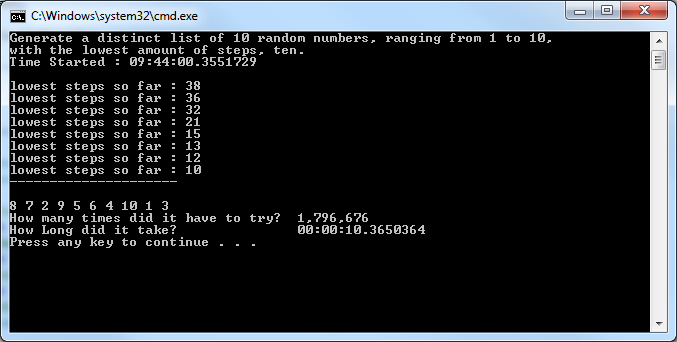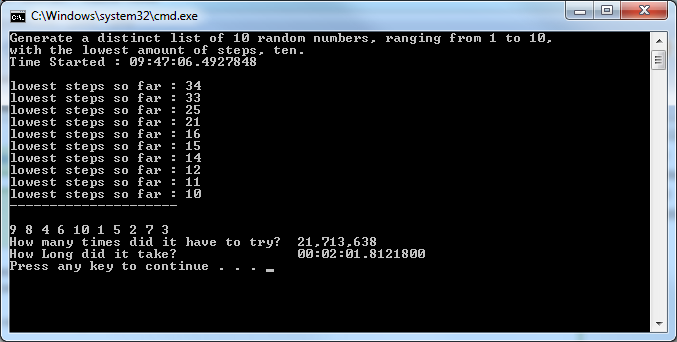# C#: Generate 10 unique integers (1-10) using only 10 steps

In this fun little program I force my computer to randomly generate a list of ten unique numbers, one through ten, using only 10 steps.

I run the program until it randomly meets all these conditions.```using System; using System.Collections.Generic; using System.Linq; using System.Text;   namespace SandCastlePractice { class Program { static void Main(string[] args) { Console.WriteLine("Generate a distinct list of 10 random numbers," +" ranging from 1 to 10, with the lowest amount of steps (10)"); DateTime timeStart = DateTime.Now; Console.WriteLine("Time Started : "+ timeStart.TimeOfDay+"\r\n");   //Counter for total times it will try int Total_Iterations = 0; //Decrementor for lowest amount of steps to create distinct list int lowestStep = 10000; //int used to see if lowest step changed int laststep = 0; //display list when lowest steps are achieved LinkedList<int> displayList = new LinkedList<int>();   //while the lowest steps arnt 10, keep trying while (lowestStep != 10) { Total_Iterations++; //steps counter int iSteps = 0; //bool to check if list of 10 is distinct bool isListDistinct = false; //temp LinkedList to be populated over and over LinkedList<int> numList = new LinkedList<int>(); //create random number object Random random = new Random(); while (!isListDistinct) { ++iSteps; //get a random number 0 - 10 int randomNumber = random.Next(0, 10); //increment it by one so its 1-10, instead of 0-9 randomNumber++; //if temp LinkedList doesn't contain generated int, add it if (!numList.Contains(randomNumber)) numList.AddLast(randomNumber); //when the list has 10 elements stop looping if (numList.Count == 10) { isListDistinct = true; //check if lowest amount of step is less then previous if (iSteps < lowestStep) lowestStep = iSteps; } } //if lowest amount of steps got lower if (laststep != lowestStep) { //Display new low Console.WriteLine("lowest steps so far : " + lowestStep); //when steps taken are 10, set current temp LinkedList //to Display List if (lowestStep == 10) displayList = numList;   } laststep = lowestStep; isListDistinct = false; }   Console.WriteLine("---------------------\r\n"); //display the distinct list of ten, that only took 10 steps to generate foreach (var num in displayList) { Console.Write(num + " "); } Console.WriteLine(); Console.WriteLine("How many times did it have to try? " + Total_Iterations.ToString("#,##0")); DateTime timeFinish = DateTime.Now; Console.WriteLine("How Long did it take? "+ (timeFinish-timeStart) ); } } }```